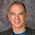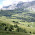## Thursday, September 5, 2013

### Clarifying Sequence of Returns Risk (Part 1)

If you read much about retirement finance, then you've probably seen a piece or two on sequence of return (SOR) risk. They don't provide much clarity, IMHO.

Different writers define SOR risk differently (part of the problem), but I use the term to describe the observation that two investors who withdraw or invest periodically from their retirement portfolio can experience the same average stock market returns and have very different outcomes when the order of those returns differs.

Those investors subject to SOR risk would include most who save in a 401(k) savings plan, investors who dollar cost average, retirees who implement a safe withdrawal rates strategy and investors who save or spend random amounts from their retirement portfolio.

In fact, as I will show, there is only one way to avoid it.

The risk is best described with an example.

I read a nice post by Dana Anspach entitled, "Sequence of Returns Risk Misunderstood by Many Retirees." It explains the effects well, except that the assertion that sequence of returns risk does not apply in the accumulation phase of retirement funding is incorrect, as I will demonstrate1.

The article shows that a series of 10 market returns:

·       1996 23.10%
·       1997 33.40%
·       1998 28.60%
·       1999 21.0%
·       2000 -9.10%
·       2001 -11.90%
·       2002 -22.10%
·       2003 28.70%
·       2004 10.90%
·       2005 4.90%

would result in a terminal portfolio value of \$164,5132 for an investor who started out with \$100,000 and spent \$6,000 per year. An investor who did exactly the same but experienced those returns in reverse order (4.9% in year one, 10.9% in year two, etc.) would end up with only \$125,691.

One thing that bothers me about this analysis, which is quite common in the literature, is that the reader may come to think that he or she is endowed with a pre-ordained set of future market returns and need only worry about their sequence.

The article compares two of these series, forward and backward, but there are actually 10!, or 3,628,800 different permutations of these ten returns (a rare opportunity to use the factorial key on my decades-old HP 12C calculator. It’s right there under the “3”.)

Retirement plans typically cover 30 years or more. How many different sequences can you create from 30 numbers? There are approximately 2.65 x 1032 permutations of 30 years of returns, or 265 followed by 30 zeroes, which rounds off to a gazillion bazillion.

These two aren’t even the best and worst series orders for the ten years of returns. The largest portfolio value will result when the returns arrive in descending order (\$181,026) and the smallest will occur when they arrive in ascending order (\$80,994).

Of course, we’re not limited to permutations of 30 or so market returns in retirement, the possibilities are limitless. So there is no “sequence” of future returns, as if when you don’t get one then you'll get the other. We have no idea what those 30 annual returns will be, so it seems pointless to hope that, whatever they might be, they arrive in decreasing order.

Sequence of returns risk isn’t a phenomenon, at all. It’s algebra.

Let’s say a retiree has a portfolio worth \$100,000 and experiences annual returns of 20% followed by a loss of 7% followed by a gain of 3%. His terminal portfolio value (TPV) can be calculated as:

We can factor out the \$100,000 initial portfolio value and get

The terminal portfolio value after three years is \$114,998. The commutative property of multiplication tells us that we will get the same result no matter what order we multiply these four factors. So, the portfolio represented by this equation will always accumulate the same total value regardless of the sequence of the returns.

If we don’t add new funds or subtract spending from our portfolio each year, there is no SOR risk.

What happens to the algebra if we do withdraw, say \$4,000 at the end of each year? The portfolio value is then calculated as follows:There are three things to observe about equation . First, the equation no longer contains only multiplication, so the commutative property does not apply and the order in which the market returns appear affects the solution. Swap the 1.2 and the 0.93 in equation , for example, and you change the result to \$101,884.

Because we are subtracting amounts each year, we now see sequence of returns risk that wasn’t present in equation .

The second thing to observe is that this would be the case if we added \$4,000 each year instead of subtracting it, so SOR risk is present in both accumulation and spending phases (just change the minus signs to plusses in equation ).

The third observation is that sequence of returns would also matter if we subtracted or added random amounts each year. SOR risk is present when we withdraw or save fixed dollar amounts periodically, as the safe withdrawal strategy requires, or if we spend or save a different dollar amount every year.

There was no SOR risk before we introduced periodic buying and/or selling from the portfolio. That tells us that SOR risk is simply the uncertainty of the stock prices at those periodic buy and sell points in our future.

Now, what happens to equation  if our investor decides to withdraw say, 4% each year of his portfolio’s current value instead of some dollar amount? Portfolio value is now calculated as:

or

which simplifies to:

Equation  now looks like equation . The percentages are each smaller by 0.04, but once again, the expression is the product of four factors, so the commutative property of multiplication applies and the sequence doesn’t matter. There is no sequence of returns risk.

Also note that if the percentage isn’t the same every year (e.g., we spend 4% of remaining portfolio value the first 5 years and 5% the second 5 years), we re-introduce sequence of returns risk.

What does this high school algebra exercise tell us about sequence of returns risk in investing for retirement?

• SOR risk is present when you invest your retirement portfolio in volatile assets like stocks. It is not present in fixed annuities and is negligible in low-volatility portfolios like short and intermediate Treasury bond ladders.

• SOR risk can be present in both accumulation and spending phases of retirement funding.

• The only way to avoid SOR risk with a risky portfolio is to withdraw the same percentage of your portfolio’s remaining balance every year.

I was exposed to SOR risk while I saved for retirement, but saving a set percentage of my increasing portfolio balance wasn’t feasible. There was no practical way to avoid SOR risk in the accumulation phase.

I withdraw about 4% of my current portfolio value every year, so I’m not exposed to SOR risk in retirement.

Lack of exposure to SOR risk did not, however, protect me from the 2007-2009 market crash (my portfolio allocation did). So, it didn't protect me from the risk that a large portfolio loss early in retirement would threaten my portfolio’s survival if I live a long life.

That’s another way financial writer’s define sequence of returns risk, but I believe that is a different risk.

I’ll tackle that in Part Two.

----------------------------

1The reader can work through the same three-year example I provide but adding a fixed dollar amount each year instead of subtracting and observe that sequence of risk does, in fact, exist in the accumulation phase.

2The referenced article states the total of \$162,548 but I believe this is in error. That number appears to be the portfolio value after 9 years, not 10. The mistake is inconsequential. I note it only to avoid confusion.

1.I agree with your statement that SOR risk is removed if you withdraw a fixed % each year. However your formula will not work because it is combining the investment return % and the withdraw % and then basing it on the portfolio value at a certain point in time. I reason that the withdrawal is on day 1 of each year, because if it is at the end of the year (day365) then the retiree would have no money for that entire year until the last day of the year. In your example, year 1 draw which is taken on the 1st day of the year is 4% based on the current value (day1) 100,000 which is fine. That would leave 96,000 (not 100,000 as in your formula) invested in year 1 to earn 20% and ending value of 115,200. On the 2nd year draw (taken out on day 1 at the beginning of Year 2, the 4% is based on what? The only current value known at that point in time is 115,200. You won't know you will earn -7% in that 2nd year until the year is over, so you can't include that in your formula. The withdrawal would be 4% of 115,200 and the ending value of year 2 will be 102,581. Finally the remaining value TPV end of year 3 would be 101,699.

1.You can withdraw money any day. You can withdraw monthly. You can withdraw weekly. I have been retired for 11 years and have never once withdrawn a year's expenses at the beginning of the year.

2.I totally Agree with you that using percentage withdrawal instead of constant dollar withdrawal (adjusted for inflation) is the way to go.

As a side note, SOR is also eliminated using any non-fixed percentage withdrawal. To have the fund at t=0 to be the same at fund t=10, you only need that the geometric average rate of return = 1/(1- geometric average rate of withdrawal).

If you go down the percentage withdrawal path, may I suggest Accumulation-Dynamic Decumulation as a strategy of withdrawal? While you get the benefit of removing SOR, you also mitigate substantially the variable withdrawal risk that you face using a percentage withdrawal. It can be used in combination with "Variable Percentage Withdrawal" or the Constant Percentage Withdrawal.

1.Thanks, Patrice. I've written about both several times. I would expand "any non-fixed percentage withdrawal" to any variable-spending strategy that reduces spending when the portfolio declines in value.

However, variable spending doesn't eliminate sequence of return risk. It simply moves SOR's effect from the probability of portfolio depletion to reduced spending, clearly the lesser of two evils.

Whether one periodically spends a fixed amount from a portfolio or a variable amount, a more favorable sequence of returns will always permit more spending.

An easy way to see this is to calculate spending for a two-year retirement with returns of +20% followed by -10% compared to returns of -10% followed by +20%. You will, in fact, end up with the same portfolio balance but you can spend more with the better sequence of returns (the first).

We're interested in spending here, not terminal portfolio value. The only way to eliminate sequence risk is to avoid periodic spending from a volatile portfolio, period. Any amount or percentage you spend will be subject to unpredictable future stock prices.